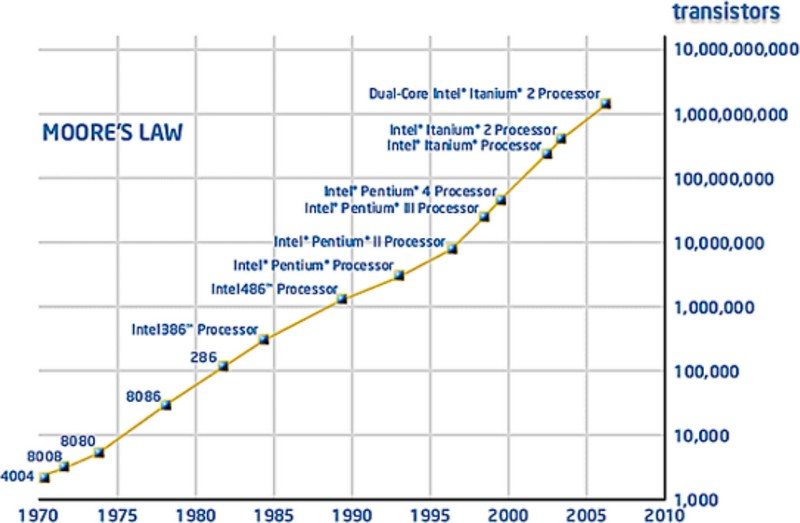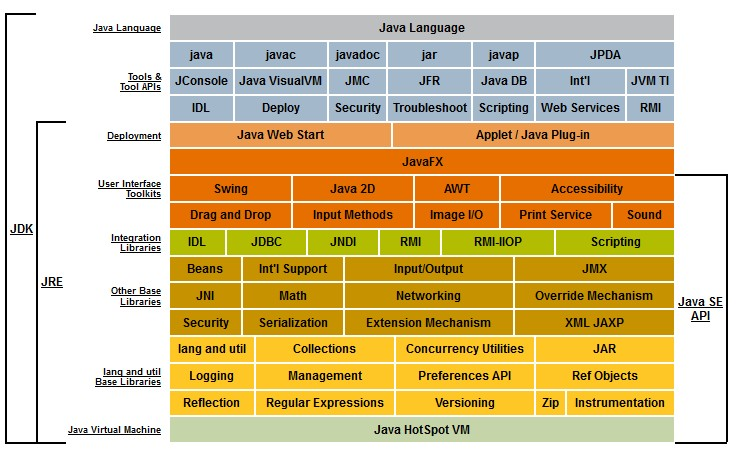A radix point (or radix character) 1) is the symbol used in numerical representations to separate:

• the integral part of a number (to the left of the radix point)
• from its fractional part (to the right of the radix point).

Not to be confound with the base also called Radix

The radix is locale dependent and is:

• a period (full stop) .,
• or a comma ,.

Recommended PagesBit - Bit Point (Or Radix Point)Decimal - Decimal Point

In base 10 notation, the radix point is more commonly called the decimal point.Fixed Point Number - Precision

Precision is the maximum number of significant digits (on either side of the radix point) in the fixed point notation The number 00123456.78 has a precision of 8 123.45 has a precision of 5...Java - Double

Double in Java They implements the 64-bit precision IEEE 754 floating point Documentation: java/lang/Double Double is a subtype of number. Literal Double.parseDouble always uses a dot...Number - Babylonian numeral system, base-60

The sexagesimal numeral system or base-60, was the first positional system in the first language developed the Cuneiform It was used by astronomers Numeral System Parts Babylonian numerals and other...Number - (Numeral system|System of numeration|Representation of number)

A numeral system (or system of numeration) is a mathematical notation system for expressing numbers using digits (or other symbols). The numeral system gives the context that allows the (digits|symbols)...Number - Fixed-point numbers (exact numbers)

A fixed-point number represents a limited-precision rational number that may have a fractional part. These numbers are stored internally in a scaled-integer form, typically in binary but sometimes in...Number - Floating-point (system|notation) - (Float|Double) - Approximate numeric

The term floating point refers to the fact that the number's radix point can float, that is, it can be placed anywhere relative to the significant digits of the number. They are fractional numbers...Number - Fraction (Fractional Number)

In number, fractional are the values posterior to the separator (radix point) 625 (i.e. 625/1000) is the fractional part to the right of the radix.Number - Integer -

An integer is the part of a number that is located at the left of the radix point. In other word, it's a number without its fractional component. Negative numbers without fractional components are...